# 1. 文本分类任务介绍

• 垃圾邮件分类：二分类问题，判断邮件是否为垃圾邮件
• 情感分析
• 二分类问题，判断文本情感是积极(positive)还是消极(negative)
• 多分类问题，判断文本情感属于{非常消极，消极，中立，积极，非常积极}中的哪一类
• 新闻主题分类：判断新闻属于哪个类别，如财经、体育、娱乐等
• 自动问答系统中的问句分类
• 社区问答系统中的问题分类：多标签分类，如知乎看山杯
• 更多应用：

• 二分类：accuracy，precision，recall，f1-score，...
• 多分类: Micro-Averaged-F1， Macro-Averaged-F1, ...
• 多标签分类：Jaccard相似系数, ...

# 2. 传统机器学习方法

• 人工降维：停用词过滤，低频n-gram过滤等
• 自动降维：LDA等

# 3. CNN用于文本分类

## 3.1 CNN模型推导

• 一个句子是由多个词拼接而成的，如果一个句子有$n$个词，且第i个词表示为$x_i$，词$x_i$通过embedding后表示为k维的向量，即$x_i\in\Re^k$，则一个句子$x_{1:n}$$n*k$的矩阵，可以形式化如下：

$X_{1:n}=x_1\oplus x_2\oplus \dots \oplus x_n$

• 一个包含$h$个的词的词窗口表示为：$$X_{i:i+h-1}\in\Re^{hk}$$
• 一个filter是大小为$h*k$的矩阵转换成的长度为$h*k$一维向量，表示为:$$W\in\Re^{hk}$$
• 通过一个filter作用一个词窗口提取可以提取一个特征$c_i$，如下：

$c_i=f(W \cdot X_{i:i+h-1}+b)其中，b\in\Re是bias值，f为激活函数如Relu等。$

$c= [c_1, c_2, \dots , c_{n-h+1}]$

• 池化操作：对一个filter提取到的feature map进行max pooling，得到$\hat{c}\in\Re$即：

$\hat{c}=max(c)$

• 若有$m$个filter，则通过一层卷积、一层池化后可以得到一个长度为$m$的向量$z\in\Re^m$:

$z = [\hat{c}_1, \hat{c}_2, \dots, \hat{c}_m]$

• 最后，将向量$z$输入到全连接层，得到最终的特征提取向量$y$ (这里的$W$为全连接层的权重，注意与filter进行区分):

$y=W \cdot z+b$

## 3.2 优化CNN模型

### 3.2.1 词向量

• 随机初始化 （CNN-rand）

• 预训练词向量进行初始化，在训练过程中固定 (CNN-static)

• 预训练词向量进行初始化，在训练过程中进行微调 (CNN-non-static)

• 多通道(CNN-multichannel):将固定的预训练词向量和微调的词向量分别当作一个通道(channel)，卷积操作同时在这两个通道上进行，可以类比于图像RGB三通道。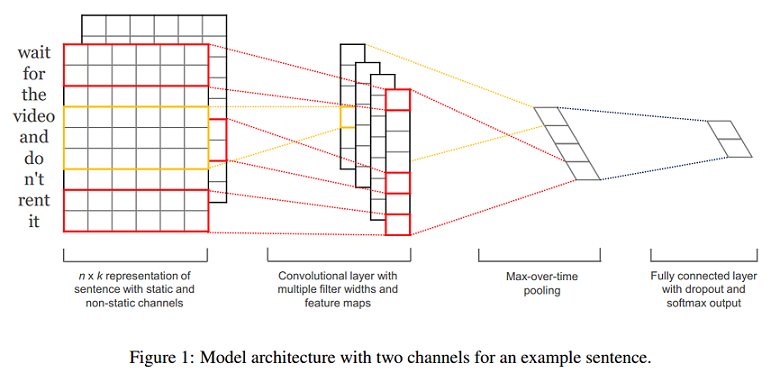• 上图为模型架构示例，在示例中，句长$n=9$，词向量维度$k=6$，filter有两种窗口大小（或者说kernel size），每种有2个，因此filter总个数$m=4$，其中:

• 一种的窗口大小$h=2$（红色框），卷积后的向量维度为$n-h+1=8$
• 另一种窗口大小$h=3$（黄色框），卷积后的向量维度为$n-h+1=7$
(论文原图中少画了一个维度，感谢@shoufengwei指正)

### 3.2.2 正则化

• Dropout: 对全连接层的输入$z$向量进行dropout

$y=W \cdot (z \circ r)+b其中r\in\Re^m为**masking**向量（每个维度值非0即1，可以通过伯努利分布随机生成），和向量z进行元素与元素对应相乘，让r向量值为0的位置对应的z向量中的元素值失效（梯度无法更新）。$

## 3.3 一些结论

• Multichannel vs. Single Channel Models: 虽然作者一开始认为多通道可以预防过拟合，从而应该表现更高，尤其是在小规模数据集上。但事实是，单通道在一些语料上比多通道更好；
• Dropout可以提高2%–4%性能(performance)；
• 对于不在预训练的word2vec中的词，使用均匀分布$U[-a,a]$随机初始化，并且调整$a$使得随机初始化的词向量和预训练的词向量保持相近的方差，可以有微弱提升；
• 可以尝试其他的词向量预训练语料，如Wikipedia[Collobert et al. (2011)]

## 3.4 进一步思考CNN

### 3.4.1 为什么CNN能够用于文本分类（NLP）？

• 为什么CNN能够用于文本分类（NLP）？
• filter相当于N-gram ？
• filter只提取局部特征？全局特征怎么办？可以融合吗？
• RNN可以提取全局特征
• RCNN（下文说明）: RNN和CNN的结合

### 3.4.2 超参数怎么调？

• 用什么样的词向量
• 使用预训练词向量比随机初始化的效果要好
• 采取微调策略（non-static）的效果比固定词向量（static）的效果要好
• 无法确定用哪种预训练词向量(Google word2vec / GloVe representations)更好，不同的任务结果不同，应该对于你当前的任务进行实验；
• filter窗口大小、数量
• 每次使用一种类型的filter进行实验，表明filter的窗口大小设置在1到10之间是一个比较合理的选择。
• 首先在一种类型的filter大小上执行搜索，以找到当前数据集的“最佳”大小，然后探索这个最佳大小附近的多种filter大小的组合。
• 每种窗口类型的filter对应的“最好”的filter个数(feature map数量)取决于具体数据集；
• 但是，可以看出，当feature map数量超过600时，performance提高有限，甚至会损害performance，这可能是过多的feature map数量导致过拟合了；
• 在实践中，100到600是一个比较合理的搜索空间。
• 激活函数 (tanh, relu, ...)
• Sigmoid, Cube, and tanh cube相较于Relu和Tanh的激活函数，表现很糟糕；
• tanh比sigmoid好，这可能是由于tanh具有zero centering property(过原点);
• 与Sigmoid相比，ReLU具有非饱和形式(a non-saturating form)的优点，并能够加速SGD的收敛。
• 对于某些数据集，线性变换(Iden，即不使用非线性激活函数)足够捕获词嵌入与输出标签之间的相关性。（但是如果有多个隐藏层，相较于非线性激活函数，Iden就不太适合了，因为完全用线性激活函数，即使有多个隐藏层，组合后整个模型还是线性的，表达能力可能不足，无法捕获足够信息）；
• 因此，建议首先考虑ReLU和tanh，也可以尝试Iden
• 池化策略：最大池化就是最好的吗
• 对于句子分类任务，1-max pooling往往比其他池化策略要好；
• 这可能是因为上下文的具体位置对于预测Label可能并不是很重要，而句子某个具体的n-gram(1-max pooling后filter提取出来的的特征)可能更可以刻画整个句子的某些含义，对于预测label更有意义；
• (但是在其他任务如释义识别，k-max pooling可能更好。)
• 正则化
• 0.1到0.5之间的非零dropout rates能够提高一些performance（尽管提升幅度很小），具体的最佳设置取决于具体数据集；
• 对l2 norm加上一个约束往往不会提高performance（除了Opi数据集）；
• 当feature map的数量大于100时，可能导致过拟合，影响performance，而dropout将减轻这种影响；
• 在卷积层上进行dropout帮助很小，而且较大的dropout rate对performance有坏的影响。

## 3.5 字符级别的CNN用于文本分类

### 3.5.1 字符级CNN的模型设计

• 定义字母表(Alphabet)：大小为$m​$ (对于英文$m=70​$，如下图，之后会考虑将大小写字母都包含在内作为对比)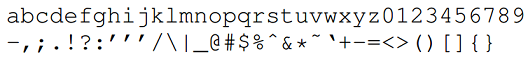• 字符数字化（编码）： "one-hot"编码
• 序列（文本）长度：$l_0$ (定值)
然后论文设计了两种类型的卷积网络：Large和Small（作为对照实验）
• 它们都有9层，其中6层为卷积层(convolutional layer)；3层为全连接层(fully-connected layer)：
• Dropout的概率都为0.5
• 使用高斯分布(Gaussian distribution)对权重进行初始化：
• 最后一层卷积层单个filter输出特征长度(the output frame length)为 $l_6 = (l_0 - 96) / 27$，推
• 第一层全连接层的输入维度(其中1024和256为filter个数或者说frame/feature size):
• Large: $l_6 * 1024$
• Small: $l_6 * 256$
• 下图为模型的一个图解示例。其中文本长度为10，第一层卷积的kernel size为3（半透明黄色正方形），卷积个数为9（Feature=9），步长为1，因此Length=10-3+1=8，然后进行非重叠的max-pooling（即pooling的stride=size），pooling size为2，因此池化后的Length = 8 / 2 = 4。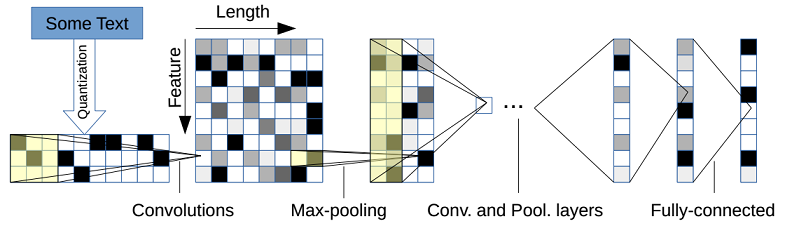### 3.5.2 字符级CNN的相关总结与思考

• 字符级CNN是一个有效的方法
• 数据集的大小可以为选择传统方法还是卷积网络模型提供指导：对于几百上千等小规模数据集，可以优先考虑传统方法，对于百万规模的数据集，字符级CNN开始表现不错。
• 字符级卷积网络很适用于用户生成数据(user-generated data)（如拼写错误，表情符号等），
• 没有免费的午餐(There is no free lunch)
• 中文怎么办
• 将字符级和词级进行结合是否结果更好
• 英文如何结合
• 中文如何结合

### 3.5.3 使用同义词表进行数据增强

• 英文同义词典: from the mytheas component used in LibreOffice1 project. http://www.libreoffice.org/
• 从给定的文本中抽取出所有可以替换的词，然后随机选择$r$个进行替换，其中$r$由一个参数为$p$的几何分布(geometric distribution)确定，即$P[r] \sim p^r$
• 给定一个待替换的词，其同义词可能有多个（一个列表），选择第$s$个的概率也通过另一个几何分布确定，即$P[s] \sim q^s$。这样是为了当前词的同义词列表中的距离较远($s$较大)的同义词被选的概率更小。
• 论文实验设置: $p=0.5, q=0.5$

# 4. RNN用于文本分类

• 策略1：直接使用RNN的最后一个单元输出向量作为文本特征
• 策略2：使用双向RNN的两个方向的输出向量的连接（concatenate）或均值作为文本特征
• 策略3：将所有RNN单元的输出向量的均值pooling或者max-pooling作为文本特征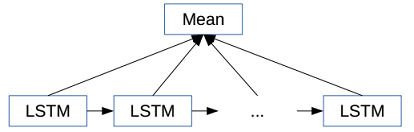• 策略4：层次RNN+Attention, Hierarchical Attention Networks

# 5. RCNN（RNN+CNN）用于文本分类

## 5.1 RCNN模型推导

### 5.1.1 词表示学习

\begin{align} c_l(w_i) = f(W^{(l)}c_l(w_{i-1})+W^{(sl)}e(w_{i-1})) ; \\ c_r(w_i) = f(W^{(r)}c_r(w_{i-1})+W^{(sr)}e(w_{i-1})) ; \\ x_i = [c_l(w_i);e(w_i);c_r(w_i)] ; \\ \end{align}

### 5.1 2 文本表示学习

• Max-pooling layer: $y^{(3)}=\max \limits_{i=1}^{n} y^{(2)}_i$
• Fully connected layer: $y^{(4)}=W^{(4)}y^{(3)}+b^{(4)}$
• Softmax layer: $p_i=\frac{\exp(y^{(4)}_i)}{\sum_{k=1}^n \exp(y^{(4)}_k)}$
下图为上述过程的一个图解: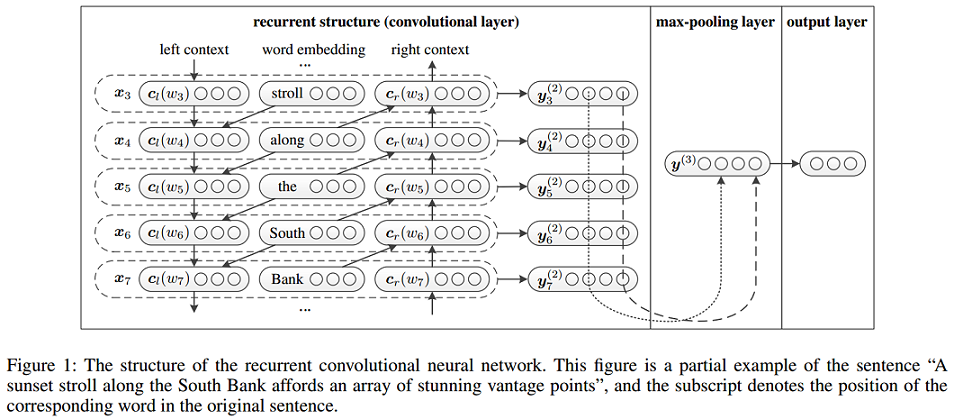## 5.2 RCNN相关总结

• NN vs. traditional methods: 在该论文的所有实验数据集上，神经网络比传统方法的效果都要好
• Convolution-based vs. RecursiveNN: 基于卷积的方法比基于递归神经网络的方法要好
• RCNN vs. CFG and C&J: The RCNN可以捕获更长的模式(patterns)
• RCNN vs. CNN: 在该论文的所有实验数据集上，RCNN比CNN更好
• CNNs使用固定的词窗口(window of words), 实验结果受窗口大小影响
• RCNNs使用循环结构捕获广泛的上下文信息

# 6. 一定要CNN/RNN吗

## 6.1 深层无序组合方法

### 6.1.1 Neural Bag-of-Words Models

• Word embedding average : $z=g(w \in X)=\frac{1}{X} \sum\limits_{w \in X} v_w$
• Softmax Layer: $\hat{y} = softmax(W_s \cdot z + b)$
• Loss function: cross-entropy error, $\iota(\hat{y}) =\sum\limits_{p=1}^{k}y_p\log(\hat{y_p})$

### 6.1.2 Considering Syntax for Composition

• Recursive neural networks (RecNNs)
• 可以考虑一些复杂的语言学现象，如否定、转折等 (优点)
• 实现效果依赖输入序列（文本）的句法树（可能不适合长文本和不太规范的文本）
• 需要更多的训练时间
• Using a convolutional network instead of a RecNN
• 时间复杂度同样比较大，甚至更大（通过实验结果得出的结论，这取决于filter大小、个数等超参数的设置）

### 6.1.3 Deep Averaging Networks

Deep Averaging Networks (DAN)是在NBOW model的基础上，通过增加多个隐藏层，增加网络的深度(Deep)。下图为带有两层隐藏层的DAN与RecNN模型的对比。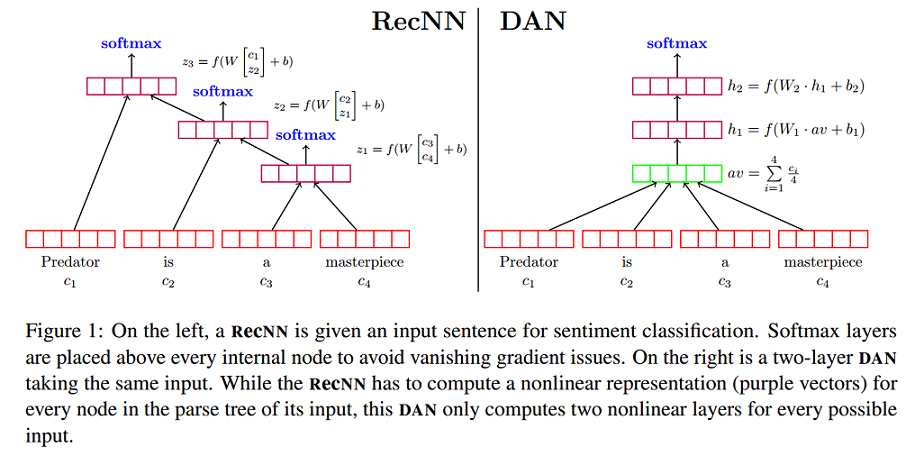### 6.1.4 Word Dropout Improves Robustness

• 针对DAN模型，论文提出一种word dropout策略：在求平均词向量前，随机使得文本中的某些单词(token)失效。形式化表示如下：

\begin{align} r_w \sim Bernoulli(p) ; \\ \hat{X} = \{w|w \in X and r_w > 0\} ; \\ z = g(w \in X ) = \frac{\sum_{w \in \hat{X}}v_w}{|\hat{X}|} ; \\ \end{align}

• Word Dropout可能会使得某些非常重要的token失效。然而，使用word dropout往往确实有提升，这可能是因为，一些对标签预测起到关键性作用的word数量往往小于无关紧要的word数量。例如，对于情感分析任务，中立(neutral)的单词往往是最多的。
• Word dropout 同样可以用于其他基于神经网络的方法。
• Word Dropout或许起到了类似数据增强(Data Augmentation)的作用?

## 6.2 fastText

### 6.2.1 fastText模型架构

fastText模型直接对所有进行embedded的特征取均值，作为文本的特征表示，如下图。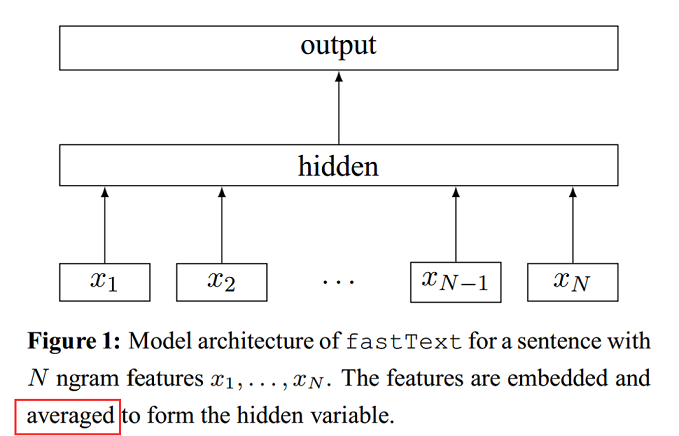### 6.2.2 特点

• 当类别数量较大时，使用Hierachical Softmax
• 将N-gram融入特征中，并且使用Hashing trick[Weinberger et al.2009]提高效率

# 7. 最新研究

References
 Le and Mikolov - 2014 - Distributed representations of sentences and documents
 Kim - 2014 - Convolutional neural networks for sentence classification
 Zhang and Wallace - 2015 - A Sensitivity Analysis of (and Practitioners' Guide to) Convolutional Neural Networks for Sentence Classification
 Zhang et al. - 2015 - Character-level convolutional networks for text classification
 Lai et al. - 2015 - Recurrent Convolutional Neural Networks for Text Classification
 Iyyer et al. - 2015 - Deep unordered composition rivals syntactic methods for Text Classification
 Joulin et al. - 2016 - Bag of tricks for efficient text classification
 Liu and Lapata - 2017 - Learning Structured Text Representations
 Yin and Schütze - 2017 - Attentive Convolution
 Zhang et al. - 2017 - Multi-Task Label Embedding for Text Classification

posted @ 2017-12-28 12:55  llhthinker  阅读(...)  评论(...编辑  收藏
TOP